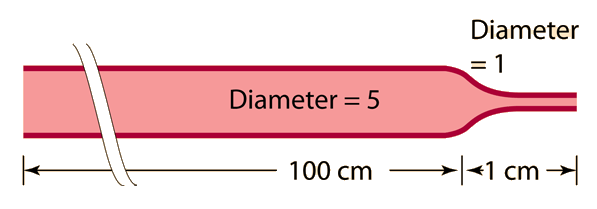# Small vessels dominate resistance

Suppose you have a vessel that is 100cm long of diameter 5, but is then constricted to diameter 1 for just one centimeter of length. How much will that constriction increase the resistance to flow?The large vessel has 100x the length, but the small vessel has 54 = 625 times the resistance per unit length. The 1 cm small section then has 6.25x the resistance of the entire length of larger vessel!

A small area of constriction in a blood vessel can make a large difference in the resistance to flow and can have major medical implications.

Resistances to fluid flow in a tube which are in series add directly, just like electrical resistances in series.

Index

Poiseuille's law concepts

 HyperPhysics***** Mechanics ***** Fluids R Nave
Go Back

# Assumptions in Fluid Calculations

In order to use Poiseuille's law in fluid calculations, it is necessary to assume that the flow is laminar and that there is no turbulence. This is often an invalid assumption since many common flow phenomena exceed the critical speeds at which turbulence begins. Even below the critical speed, the existence of irregularities, angular junctions, or obstructions will produce turbulence. When the Bernoulli equation is used to calculate pressures and velocities in constrictions, similar assumptions are made. Even though the Bernoulli equation (being a statement of conservation of energy) remains valid in the case of turbulence, not all the fluid kinetic energy is contributing to the volume flowrate - the effective velocity gained from the fluid kinetic energy cannot be use in the expression "area x velocity = volume flowrate" since part of the kinetic energy is that of random, chaotic motion. Finally, the rms velocity calculated from the velocity profile of the laminar flow is used in the calculation of fluid kinetic energy in the Bernoulli equation applications.
Index

Poiseuille's law concepts

 HyperPhysics***** Mechanics ***** Fluids R Nave
Go Back